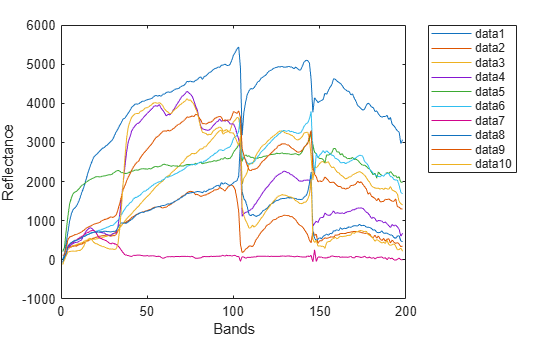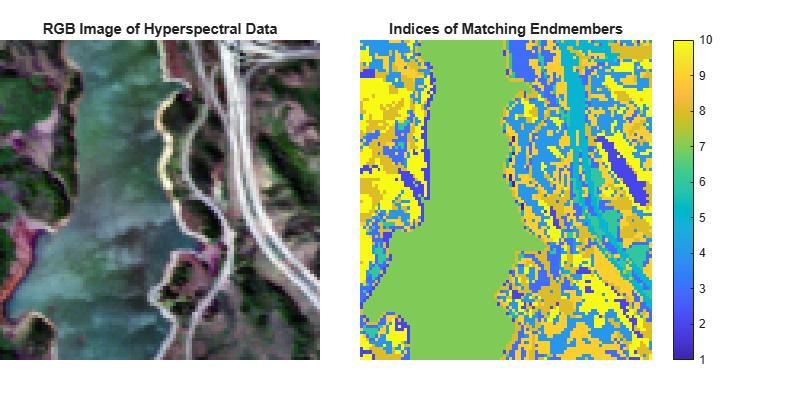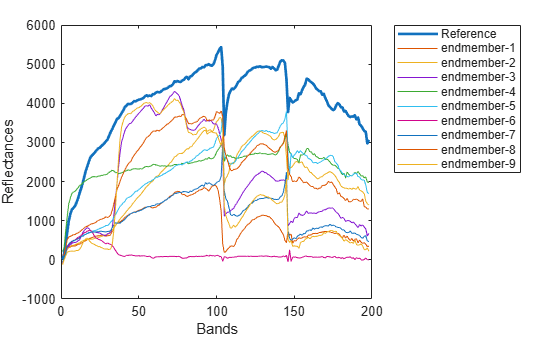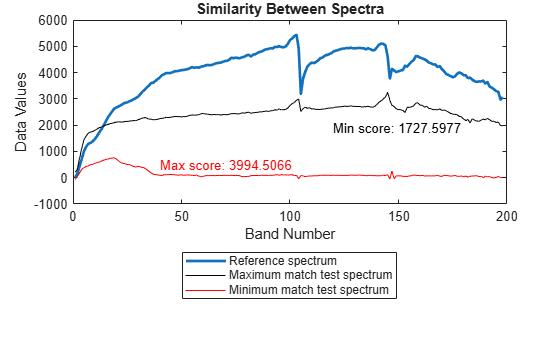# ns3

Measure normalized spectral similarity score

Since R2020b

## Syntax

``score = ns3(inputData,refSpectrum)``
``score = ns3(testSpectrum,refSpectrum)``

## Description

example

````score = ns3(inputData,refSpectrum)` measures the spectral similarity between the spectrum of each pixel in the hyperspectral data `inputData` and the specified reference spectrum `refSpectrum` by using the normalized spectral similarity score (NS3) method. Use this syntax to identify different regions or materials in a hyperspectral data cube. For information about the NS3 method, see More About.```

example

````score = ns3(testSpectrum,refSpectrum)` measures the spectral similarity between the specified test spectrum `testSpectrum` and reference spectrum `refSpectrum` by using the NS3 method. Use this syntax to compare the spectral signature of an unknown material against the reference spectrum or to compute spectral variability between two spectral signatures. NoteThis function requires the Image Processing Toolbox™ Hyperspectral Imaging Library. You can install the Image Processing Toolbox Hyperspectral Imaging Library from Add-On Explorer. For more information about installing add-ons, see Get and Manage Add-Ons.The Image Processing Toolbox Hyperspectral Imaging Library requires desktop MATLAB®, as MATLAB Online™ or MATLAB Mobile™ do not support the library. ```

## Examples

collapse all

Read hyperspectral data into the workspace.

`hcube = hypercube('jasperRidge2_R198.hdr');`

Estimate the number of spectrally distinct endmembers in the data cube by using `countEndmembersHFC` function.

`numEndmembers = countEndmembersHFC(hcube,'PFA',10^-7);`

Extract the endmember spectral signatures from the data cube by using the NFINDR algorithm.

`endmembers = nfindr(hcube,numEndmembers);`

Plot the spectral signatures of the extracted endmembers.

```figure plot(endmembers) xlabel('Bands') ylabel('Reflectance') legend('Location','Bestoutside')```Compute the NS3 distance between each endmember and the spectrum of each pixel in the data cube.

```score = zeros(size(hcube.DataCube,1),size(hcube.DataCube,2),numEndmembers); for i = 1:numEndmembers score(:,:,i) = ns3(hcube,endmembers(:,i)); end```

Compute the minimum score value from the distance scores obtained for each pixel spectrum with respect to all the endmembers. The index of each minimum score identifies the endmember spectrum to which a pixel spectrum exhibits maximum similarity. An index value, n, at the spatial location (x, y) in the score matrix indicates that the spectral signature of the pixel at spatial location (x, y) in the data cube best matches the spectral signature of the nth endmember.

`[~,matchingIdx] = min(score,[],3);`

Estimate an RGB image of the input data by using the `colorize` function.

`rgbImg = colorize(hcube,'Method','rgb','ContrastStretching',true);`

Display both the RGB image and the matrix of matched index values.

```figure('Position',[0 0 800 400]) subplot('Position',[0 0.1 0.4 0.8]) imagesc(rgbImg) axis off title('RGB Image of Hyperspectral Data') subplot('Position',[0.45 0.1 0.45 0.8]) imagesc(matchingIdx) axis off title('Indices of Matching Endmembers') colorbar```Read hyperspectral data into the workspace.

`hcube = hypercube('jasperRidge2_R198.hdr');`

Find the first 10 endmembers of the hyperspectral data.

```numEndmembers = 10; endmembers = nfindr(hcube,numEndmembers);```

Consider the first endmember as the reference spectrum and the rest of the endmembers as the test spectrum.

```refSpectrum = endmembers(:,1); testSpectra = endmembers(:,2:end);```

Plot the reference spectrum and other endmember spectra.

```figure plot(refSpectrum,'LineWidth',2) hold on plot(testSpectra) hold off label = cell(1,numEndmembers-1); label{1} = 'Reference'; for itr = 1:numEndmembers-1 label{itr+1} = ['endmember-' num2str(itr)]; end xlabel('Bands') ylabel('Reflectances') legend(label,'Location','Bestoutside')```Compute the NS3 score between the reference and test spectra.

```score = zeros(1,numEndmembers-1); for itr = 1:numEndmembers-1 score(itr) = ns3(testSpectra(:,itr),refSpectrum); end```

Find the test spectrum that exhibit maximum similarity (minimum distance) to the reference spectrum. Then, find the test spectrum that exhibit minimum similarity (maximum distance) to the reference spectrum.

```[minval,minidx] = min(score); maxMatch = testSpectra(:,minidx); [maxval,maxidx] = max(score); minMatch = testSpectra(:,maxidx);```

Plot the reference spectrum, the maximum similarity, and the minimum similarity test spectrum. The test spectrum with the minimum score value indicates highest similarity to the reference endmember. On the other hand, the test spectrum with the maximum score value has the highest spectral variability.

```figure plot(refSpectrum,'LineWidth',2) hold on plot(maxMatch,'k') plot(minMatch,'r') xlabel('Band Number') ylabel('Data Values') legend('Reference spectrum','Maximum match test spectrum','Minimum match test spectrum',... 'Location','Southoutside') title('Similarity Between Spectra') text(40,500,['Max score: ' num2str(maxval)],'Color','r') text(120,1900,['Min score: ' num2str(minval)],'Color','k')```## Input Arguments

collapse all

Input hyperspectral data, specified as a `hypercube` object or a 3-D numeric array containing the data cube. If the input is a `hypercube` object, the data is read from the `DataCube` property of the object.

Test spectrum, specified as a C-element vector. The test spectrum is the spectral signature of an unknown region or material.

Data Types: `single` | `double` | `int8` | `int16` | `int32` | `int64` | `uint8` | `uint16` | `uint32` | `uint64`

Reference spectrum, specified as a C-element vector. The reference spectrum is the spectral signature of a known region or material. The function matches the test spectrum against these values.

Data Types: `single` | `double` | `int8` | `int16` | `int32` | `int64` | `uint8` | `uint16` | `uint32` | `uint64`

## Output Arguments

collapse all

NS3 score, returned as a scalar or matrix. The output is a

• scalar — If you specify the `testSpectrum` input argument. The function matches the test spectral signature against the reference spectral signature and returns a scalar value. Both the test and the reference spectra must be vectors of same length.

• matrix — If you specify the `inputData` input argument. The function matches the spectral signature of each pixel in the data cube against the reference spectral signature and returns a matrix. If the data cube is of size M-by-N-by-C and the reference spectrum is a vector of length C, the output matrix is of size M-by-N.

A smaller NS3 score indicates a strong match between the test signature and the reference signature.

Data Types: `single` | `double`

## Limitations

This function does not support `parfor` loops, as its performance is already optimized. (since R2023a)

collapse all

### Normalized Spectral Similarity Score (NS3)

The NS3 method computes spectral similarity based on the Euclidean and SAM distances between two spectra. Let r and t be the reference and test spectra respectively. Compute the Euclidean distance between two spectra as:

`${A}_{Euclidean}=\text{\hspace{0.17em}}\sqrt{\frac{1}{C}\sum _{i=1}^{C}{\left({t}_{i}-{r}_{i}\right)}^{2}}$`

Then, compute the SAM value α

`$\alpha =\text{\hspace{0.17em}}{\mathrm{cos}}^{-1}\left(\frac{\sum _{i=1}^{C}{t}_{i}{r}_{i}}{\sqrt{\sum _{i=1}^{C}{t}_{i}^{2}}\sqrt{\sum _{i=1}^{C}{r}_{i}^{2}}}\right).$`

Finally, compute the NS3 score as:

`$NS3\text{\hspace{0.17em}}=\text{\hspace{0.17em}}\sqrt{{A}_{Euclidean}{}^{2}+{\left(1-\mathrm{cos}\left(\alpha \right)\right)}^{2}}$`

 Nidamanuri, Rama Rao, and Bernd Zbell. “Normalized Spectral Similarity Score (NS3) as an Efficient Spectral Library Searching Method for Hyperspectral Image Classification.” IEEE Journal of Selected Topics in Applied Earth Observations and Remote Sensing 4, no. 1 (March 2011): 226–40. https://doi.org/10.1109/JSTARS.2010.2086435.